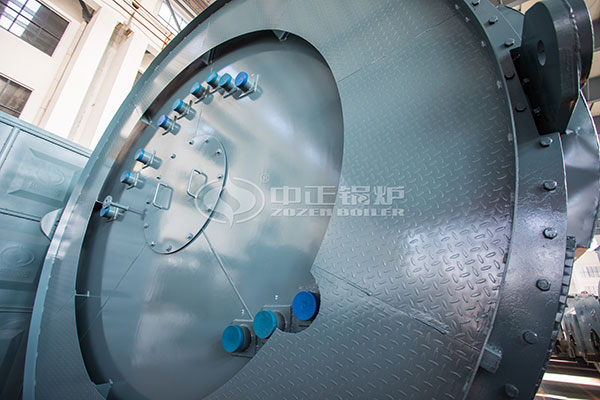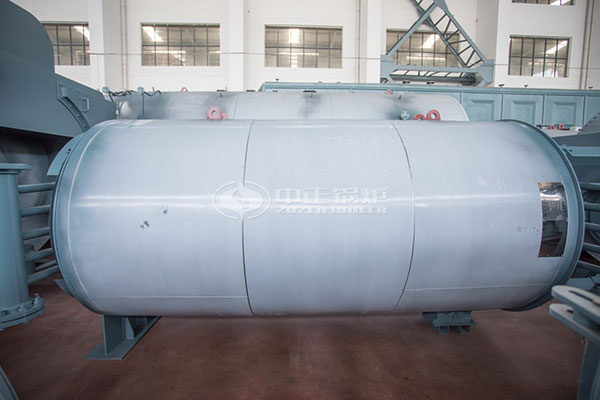# Average Cost of High Efficiency Gas Boiler

March 19, 2020 Tags:

Many manufacturing industries are inseparable from boilers, among which the most widely used are oil and gas boilers. However, with the increasing global environmental pollution, the country has also set standards for pollution emissions from various industries, which uses energy efficiently while saving energy. Reduction of emissions has become very important, so how much natural gas does a 4-ton gas boiler burn per day? How to calculate the daily operating cost of a 4-ton gas boiler?Calculation of gas boiler gas consumption

Calculation formula: gas boiler gas consumption (per hour) = gas boiler power * time / fuel heating value / thermal efficiency.

According to the non-stop operation 24 hours a day, multiplying the result of the above calculation formula by 24 hours is the total gas consumption of a 4-ton gas boiler.

The following is a detailed calculation based on the specific rated power of 2.8MW and the heating value of the fuel of 35.53MJ / Nm3:

Assume that the gas boiler thermal efficiency is 88%. Then, the gas consumption per hour of a 4-ton gas boiler = 2.8MW * 3600s / 35.53MJ / Nm3 / 88% = 320m3 / h.

It operates 24 hours a day and consumes natural gas = 320 * 24 = 7680 cubic meters.4 ton boiler daily operation cost

Natural gas prices vary in various parts of the country. The general price range is between 1.8-5 yuan. If it is for industrial use, the sales price of natural gas is generally 1.8-3 yuan per cubic meter. According to this calculation, the daily consumption of the 4-ton gas boiler is calculated. Gas volume 7680 cubic meters * (1.8-3 yuan) = 13824-23040 yuan.

How gas boilers are more energy efficient

1. Insulation, energy-saving of gas boilers starts from various parts of the boiler. For example, from the insulation of the boiler, the outer temperature of the boiler must not be too high, generally below 50 degrees Celsius.

2. Energy saver, gas boiler If you want to save costs to maximize the efficiency of the boiler, you can install a boiler energy saver to reduce the boiler exhaust temperature and make good use of the waste heat.

3. Thermostat, gas hot water boiler can be installed with a thermostat, increasing the return water temperature can also play a good energy saving effect.